Assertion & Reason Test: Coordinate Geometry - 2

# Assertion & Reason Test: Coordinate Geometry - 2

Test Description

## 10 Questions MCQ Test Online MCQ Tests for Class 10 | Assertion & Reason Test: Coordinate Geometry - 2

Assertion & Reason Test: Coordinate Geometry - 2 for Class 10 2022 is part of Online MCQ Tests for Class 10 preparation. The Assertion & Reason Test: Coordinate Geometry - 2 questions and answers have been prepared according to the Class 10 exam syllabus.The Assertion & Reason Test: Coordinate Geometry - 2 MCQs are made for Class 10 2022 Exam. Find important definitions, questions, notes, meanings, examples, exercises, MCQs and online tests for Assertion & Reason Test: Coordinate Geometry - 2 below.
Solutions of Assertion & Reason Test: Coordinate Geometry - 2 questions in English are available as part of our Online MCQ Tests for Class 10 for Class 10 & Assertion & Reason Test: Coordinate Geometry - 2 solutions in Hindi for Online MCQ Tests for Class 10 course. Download more important topics, notes, lectures and mock test series for Class 10 Exam by signing up for free. Attempt Assertion & Reason Test: Coordinate Geometry - 2 | 10 questions in 20 minutes | Mock test for Class 10 preparation | Free important questions MCQ to study Online MCQ Tests for Class 10 for Class 10 Exam | Download free PDF with solutions
 1 Crore+ students have signed up on EduRev. Have you?
Assertion & Reason Test: Coordinate Geometry - 2 - Question 1

### Directions: In the following questions, A statement of Assertion (A) is followed by a statement of Reason (R). Mark the correct choice as.Assertion : The point (-1, 6) divides the line segment joining the points (-3, 10) and (6, -8) in the ratio 2 : 7 internally.Reason : Three points A,B and C are collinear if AB + BC = AC

Detailed Solution for Assertion & Reason Test: Coordinate Geometry - 2 - Question 1 We know that the three points A,B and C are collinear if AB + BC = AC

So, Reason is correct.

Let the ratio is k : 1. Here, x1 = -3, y1 = 10, x2 = 6, y2 = -8, x = -1, y = 6

Now, y-coordinate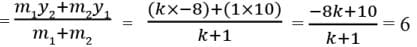⇒ -8k + 10 = 6k + 6

⇒ 10 – 6 = 6k + 8k

⇒ 14k = 4

⇒ k = 4/14 = 2/7

So, Assertion is correct

But reason (R) is not the correct explanation of assertion (A).

Assertion & Reason Test: Coordinate Geometry - 2 - Question 2

### Directions: In the following questions, A statement of Assertion (A) is followed by a statement of Reason (R). Mark the correct choice as.Assertion : The point (-1, 6) divides the line segment joining the points (-3, 10) and (6, -8) in the ratio 2 :7 internally.Reason : Three points A,B and C are collinear if area of △ ABC = 0 .

Detailed Solution for Assertion & Reason Test: Coordinate Geometry - 2 - Question 2 Using section formula, we have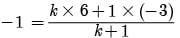- k- 1 = 6k -3

7k = 2

k = 2/7

Ratio be 2 :7 internally.

Also, if ar(△ ABC) = 0

A,B and C all these points are collinear.

Assertion & Reason Test: Coordinate Geometry - 2 - Question 3

### Directions: In the following questions, A statement of Assertion (A) is followed by a statement of Reason (R). Mark the correct choice as.Assertion : The value of y is 6, for which the distance between the points P(2,- 3) and Q(10, y) is 10.Reason : Distance between two given points A(x1, y1) and B(x2, y2) is given by,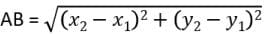Detailed Solution for Assertion & Reason Test: Coordinate Geometry - 2 - Question 3 We know that the Distance between two given points A(x1, y1) and B(x2, y2) is given by,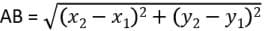So, Reason is correct.

Now, PQ = 10 ⇒ PQ2 = 100

⇒ (10 – 2)2 + (y + 3)2 = 100

⇒ (y + 3)2 = 100 – 64 = 36

⇒ y + 3 = ±6

⇒ y = -3 ± 6 ⇒ y = 3, -9

So, Assertion is not correct

Assertion & Reason Test: Coordinate Geometry - 2 - Question 4

Directions: In the following questions, A statement of Assertion (A) is followed by a statement of Reason (R). Mark the correct choice as.

Assertion : If A (2a, 4a) and B (2a, 6a) are two vertices of a equilateral triangle ABC then the vertex C is given by (2a + a√3, 5a).

Reason : In equilateral triangle all the coordinates of three vertices can be rational.

Detailed Solution for Assertion & Reason Test: Coordinate Geometry - 2 - Question 4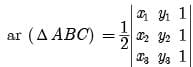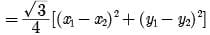LHS = rational

RHS = irrational

Hence, (x1, y1) (x2, y2) and (x3, y3) cannot be all rational.

Assertion & Reason Test: Coordinate Geometry - 2 - Question 5

Directions: In the following questions, A statement of Assertion (A) is followed by a statement of Reason (R). Mark the correct choice as.

Assertion : The point (0, 4) lies on y -axis.

Reason : The x co-ordinate on the point on y -axis is zero.

Detailed Solution for Assertion & Reason Test: Coordinate Geometry - 2 - Question 5 We know that the if the point lies on y-axis, its x-coordinate is 0.

So, Reason is correct.

The x co-ordinate of the point (0, 4) is zero.

So, Point (0, 4) lies on y -axis.

So, Assertion is also correct

Assertion & Reason Test: Coordinate Geometry - 2 - Question 6

Directions: In the following questions, A statement of Assertion (A) is followed by a statement of Reason (R). Mark the correct choice as.

Assertion : The value of y is 6, for which the distance between the points P(2, -3) and Q(10, y) is 10.

Reason : Distance between two given points A (x1, y1) and B(x2, y2) is given 6,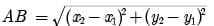Detailed Solution for Assertion & Reason Test: Coordinate Geometry - 2 - Question 6

PQ = 10

PQ2 = 100

(10 - 2)2 + (y + 3)2 = 100

(y + 3)2 = 100 - 64 = 36

y + 3 = + 6

y = - 3 + 6

y = 3, -9

Assertion & Reason Test: Coordinate Geometry - 2 - Question 7

Directions: In the following questions, A statement of Assertion (A) is followed by a statement of Reason (R). Mark the correct choice as.

Assertion : C is the mid-point of PQ, if P is (4, x), C is (y,- 1) and Q is (- 2, 4), then x and y respectively are -6 and 1.

Reason : The mid-point of the line segment joining the points P(x1, y1) and Q(x2, y2) is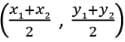Detailed Solution for Assertion & Reason Test: Coordinate Geometry - 2 - Question 7 We know that the mid-point of the line segment joining the points P(x1, y1) and Q(x2, y2) is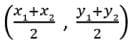So, Reason is correct.

Since, C(y,- 1) is the mid-point of P(4, x) and Q(- 2, 4).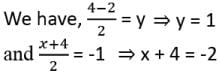⇒ x = -6

So, Assertion is correct

Assertion & Reason Test: Coordinate Geometry - 2 - Question 8

Directions: In the following questions, A statement of Assertion (A) is followed by a statement of Reason (R). Mark the correct choice as.

Assertion : The point (0, 4) lies on y -axis.

Reason : The x co-ordinate on the point on y -axis is zero.

Detailed Solution for Assertion & Reason Test: Coordinate Geometry - 2 - Question 8 The x co-ordinate of the point (0, 4) is zero.

Point (0, 4) lies on y -axis.

Assertion & Reason Test: Coordinate Geometry - 2 - Question 9

Directions: In the following questions, A statement of Assertion (A) is followed by a statement of Reason (R). Mark the correct choice as.

Assertion : Ratio in which the line 3x + 4y = 7 divides the line segment joining the points (1, 2) and (- 2, 1) is 3 : 5Reason : The coordinates of the point P(x, y) which divides the line segment joining the points A(x1, y1) and B(x2, y2) in the ratio m1 : m2 is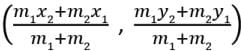Detailed Solution for Assertion & Reason Test: Coordinate Geometry - 2 - Question 9 We know that the coordinates of the point P(x, y) which divides the line segment joining the points A(x1, y1) and B(x2, y2) in the ratio m1 : m2 is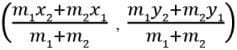So, Reason is correct.

Let the ratio is k : 1.

Here, x1 = 1, y1 = 2, x2 = -2, y2 = 1, m1 = k, m2 = 1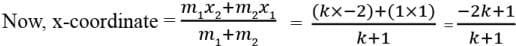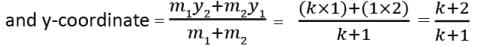Now, 3x + 4y = 7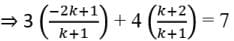⇒ 7k + 2k = 11 – 7 ⇒ 9k = 4 ⇒ k = 4/9

So, Assertion is not correct

Assertion & Reason Test: Coordinate Geometry - 2 - Question 10

Directions: In the following questions, A statement of Assertion (A) is followed by a statement of Reason (R). Mark the correct choice as.

Assertion: The point which divides the line joining the points A(1, 2) and B(- 1, 1) internally in the ratio 1: 2 is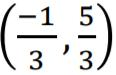Reason : The coordinates of the point P(x, y) which divides the line segment joining the points A(x1, y1) and B(x2, y2) in the ratio m1 : m2 is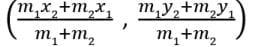Detailed Solution for Assertion & Reason Test: Coordinate Geometry - 2 - Question 10

We know that the coordinates of the point P(x, y) which divides the line segment joining the points A(x1, y1) and B(x2, y2) in the ratio m1 : m2 is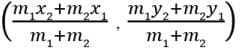So, Reason is correct.

Here, x1 = 1, y1 = 2, x2 = -1, y2 = 1, m1 = 1, m2 = 2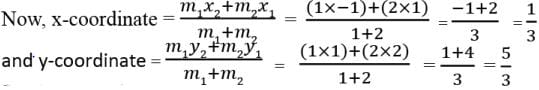So, Assertion is correct.

## Online MCQ Tests for Class 10

456 tests
 Use Code STAYHOME200 and get INR 200 additional OFF Use Coupon Code
Information about Assertion & Reason Test: Coordinate Geometry - 2 Page
In this test you can find the Exam questions for Assertion & Reason Test: Coordinate Geometry - 2 solved & explained in the simplest way possible. Besides giving Questions and answers for Assertion & Reason Test: Coordinate Geometry - 2, EduRev gives you an ample number of Online tests for practice

456 tests